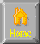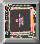```CHAOS THEORY - CRITICAL POINTS

+Critical points

a>0 no critical pts
|  |  a=0 degenerate critical pts
._--_.  |  |   |     a<0 2 nondegen critical pts
/      \ | /   /     /      (saddle function)
/        \|/   /     /
---------/----------+---------/------------
/     /    /|\       /
/     /    / | \     /
/     |    |  |  -___-
/     /     |  |    ^
/               |    |
Critical point - equilibrium pt = attractor

family f(x) = x^3/3 + ax
x^3/3 = degenerate critical point
ax    = stabilizer (polynomial)

+Local/Global Behavior
Local Infor @ critical point -> global behavior

|
@@@@@@  |               Information of behavior near
/      \ |           /   critical points (@@@@@) Gives  /
/        \|          /    global behavior of function ( / )
---------/----------+---------/------------                      /
/           |\       /
/            | \     /
/             |  @@@@@
|
|
Critical point -> dominant terms of taylor expansion @ x0
Critical point (singularity) attractor/repellor / lorenz shifter

+Whitney's theorem (hassler whitney 1955)
any smooth transformation (w/ no creases taking points
of a plane into another plane , only types of points are regular pt, folds, cusps

++Morse Theorem (marston Morse 1935)
A morse function ( = function w/ no degenerate critical points)
near a critical point of index k (=# dimensions that bend up)
there is a smooth change of coordinates  s.t. the tayl of the
taylor series expansion of f vanishes (diffeomorphism
= smooth equivalence)
f(x)=a0(xo) + [a1(xo)](x-x0) + [a2(xo)](x-x0)^2 + ...
f'(x)  (    )    f''(x) (    )   + tayl
Tayl = higher order terms of first non zero a factor
1. Small perturbation of a morse function = another morse function
2. degenerate ritical pt xform into nondegenerate critical pt w/ small "jiggle " of original function

+Splitting Lemma non-morse function f=p(y)+Q(y) ; p(y) = non morse order 3+, q(y)=morse quadratic

+Thom Classification Theorem

corank
/codim fn fold      name               spatial          temporal
1/1    y^3          fold               boundary         begin/end
1/2    y^4          cusp               pleat,fault      separate/unite,change
1/3    y^5          swallowtail        split,furrow     split;tear
1/4    y^6          butterfly          pocket           give/rcv;fill/empty
2/3    y1^3-3y1y2^2 elliptic umbilic   wave crest,arch  collapse engulf
2/3    y1^3+y2^3    hyperbolic umbilic spike,hair       drill
2/4    y1^2y2+y2^4  parabolic umbilic  mouth            open/close,eject

+corank = degree of independence = # of vanishings in 2nd order in taylor's expansion
+codimension (morse function ness)minimal # of independent terms to add to make non-morse function into morse
+Catastrophe theory( 1970 thom)
becoming degenerate critical point =
bifurcation = catastrophe pt = lorenz shift pt (equilib shift)=
discoherent / discontinuous jump (stock boom/bust,morpheogenesis,origami fold)

1) Morpheogenesis
2) 11/1940 Tacoma Narrow Bridge

```HomeNext ... Computation